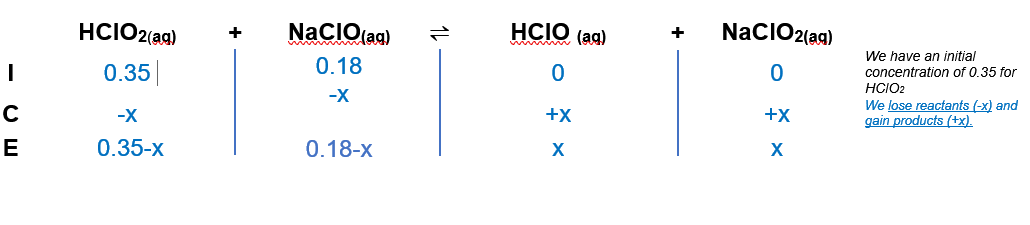# Problem: To 1.0 L of a 0.35 M solution of HClO2 is added 0.18 mol of NaClO.Calculate the [HClO2] at equilibrium.

###### FREE Expert Solution

We’re being asked to calculate the [HClO2] at equilibrium when 1.0 L of a 0.35 M solution of HClOis added 0.18 mol of NaClO.

Since HClOis a weak acid it will not dissociate completely in the aqueous solution and NaClO.

Remember that weak acids partially dissociate in water and that acids donate H+ to the base (water in this case). Therefore, the equilibrium constants for the following reactions are as follows:

HClO2(aq)  H+(aq) + ClO2(aq);                  Ka1 = 1.1 × 10–2 (can be found in books and the internet)

ClO- (aq) + H3O+(aq) ⇌ HClO(aq) + H2O (l); Ka = 2.9 x 10-8 (can be found in books and the internet)

The overall reaction is:

HClO2 (aq) +  ClO- (aq) HClO (aq) + ClO2- (aq)

From this, we can construct an ICE table. Remember that liquids are ignored in the ICE table.The Ka expression for the reaction is:

Liquids are ignored in the Kexpression.

Note that each concentration is raised by the stoichiometric coefficient: [HClO2], [NaClO2], [NaClO] and [HClO] are raised to 1.

98% (100 ratings)###### Problem Details

To 1.0 L of a 0.35 M solution of HClO2 is added 0.18 mol of NaClO.

Calculate the [HClO2] at equilibrium.